### Example 7.3 Model for Series J Data from Box and Jenkins

This example uses the Series J data from Box and Jenkins (1976). First, the input series `X` is modeled with a univariate ARMA model. Next, the dependent series `Y` is cross-correlated with the input series. Since a model has been fit to `X`, both `Y` and `X` are prewhitened by this model before the sample cross-correlations are computed. Next, a transfer function model is fit with no structure on the noise term. The residuals from this model are analyzed; then, the full model, transfer function and noise, is fit to the data.

The following statements read `Input Gas Rate` and ```Output CO``` from a gas furnace. (Data values are not shown. The full example including data is in the SAS/ETS sample library.)

```title1 'Gas Furnace Data';
title2 '(Box and Jenkins, Series J)';
data seriesj;
input x y @@;
label x = 'Input Gas Rate'
y = 'Output CO2';
datalines;
-0.109  53.8  0.000  53.6  0.178  53.5  0.339  53.5

... more lines ...

```

The following statements produce Output 7.3.1 through Output 7.3.11:

```proc arima data=seriesj;

/*--- Look at the input process ----------------------------*/
identify var=x;
run;

/*--- Fit a model for the input ----------------------------*/
estimate p=3 plot;
run;

/*--- Crosscorrelation of prewhitened series ---------------*/
identify var=y crosscorr=(x) nlag=12;
run;

/*--- Fit a simple transfer function - look at residuals ---*/
estimate input=( 3 \$ (1,2)/(1) x );
run;

/*--- Final Model - look at residuals ----------------------*/
estimate p=2 input=( 3 \$ (1,2)/(1) x );
run;

quit;
```

The results of the first IDENTIFY statement for the input series `X` are shown in Output 7.3.1. The correlation analysis suggests an AR(3) model.

Output 7.3.1: IDENTIFY Statement Results for X

 Gas Furnace Data (Box and Jenkins, Series J)

The ARIMA Procedure

Name of Variable = x
Mean of Working Series -0.05683
Standard Deviation 1.070952
Number of Observations 296

Output 7.3.2: IDENTIFY Statement Results for X: Trend and Correlation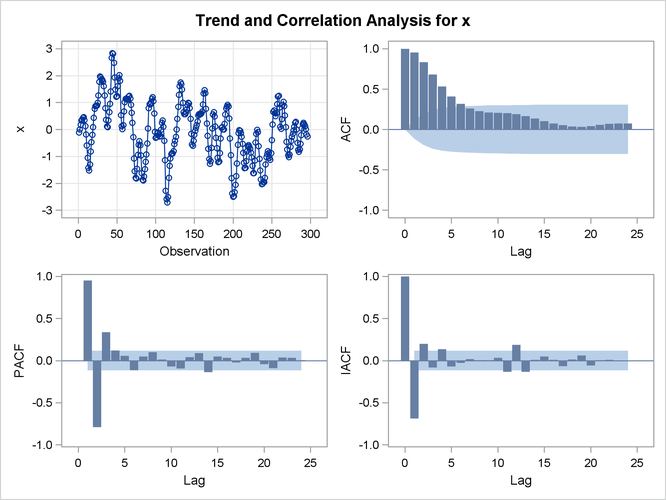The ESTIMATE statement results for the AR(3) model for the input series `X` are shown in Output 7.3.3.

Output 7.3.3: Estimates of the AR(3) Model for X

Conditional Least Squares Estimation
Parameter Estimate Standard Error t Value Approx
Pr > |t|
Lag
MU -0.12280 0.10902 -1.13 0.2609 0
AR1,1 1.97607 0.05499 35.94 <.0001 1
AR1,2 -1.37499 0.09967 -13.80 <.0001 2
AR1,3 0.34336 0.05502 6.24 <.0001 3

 Constant Estimate -0.00682 0.035797 0.1892 -141.667 -126.906 296

Model for variable x
Estimated Mean -0.1228

Autoregressive Factors
Factor 1: 1 - 1.97607 B**(1) + 1.37499 B**(2) - 0.34336 B**(3)

The IDENTIFY statement results for the dependent series Y cross-correlated with the input series `X` are shown in Output 7.3.4, Output 7.3.5, Output 7.3.6, and Output 7.3.7. Since a model has been fit to `X`, both `Y` and `X` are prewhitened by this model before the sample cross-correlations are computed.

Output 7.3.4: Summary Table: Y Cross-Correlated with X

Correlation of y and x
Number of Observations 296
Variance of transformed series y 0.131438
Variance of transformed series x 0.035357

Both series have been prewhitened.

Output 7.3.5: Prewhitening Filter

Autoregressive Factors
Factor 1: 1 - 1.97607 B**(1) + 1.37499 B**(2) - 0.34336 B**(3)

Output 7.3.6: IDENTIFY Statement Results for Y: Trend and Correlation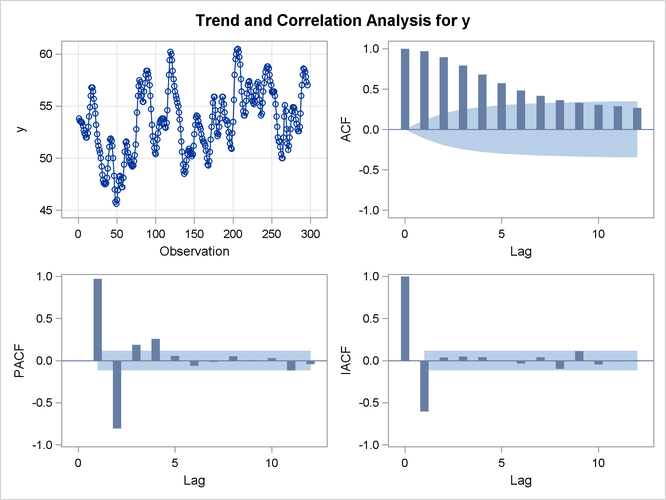Output 7.3.7: IDENTIFY Statement for Y Cross-Correlated with X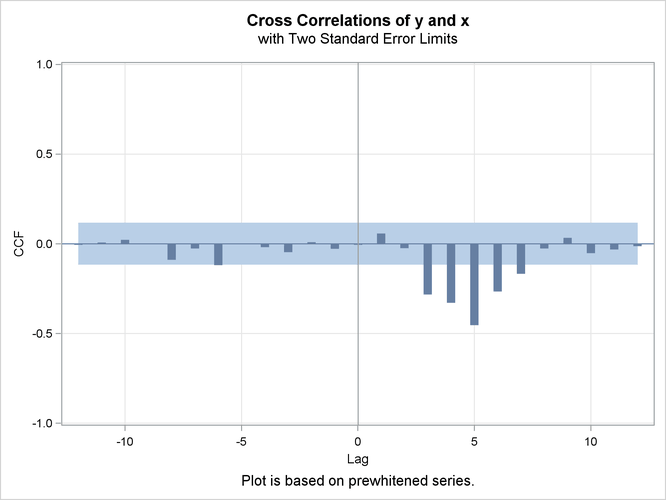The ESTIMATE statement results for the transfer function model with no structure on the noise term are shown in Output 7.3.8, Output 7.3.9, and Output 7.3.10.

Output 7.3.8: Estimation Output of the First Transfer Function Model

Conditional Least Squares Estimation
Parameter Estimate Standard Error t Value Approx
Pr > |t|
Lag Variable Shift
MU 53.32256 0.04926 1082.51 <.0001 0 y 0
NUM1 -0.56467 0.22405 -2.52 0.0123 0 x 3
NUM1,1 0.42623 0.46472 0.92 0.3598 1 x 3
NUM1,2 0.29914 0.35506 0.84 0.4002 2 x 3
DEN1,1 0.60073 0.04101 14.65 <.0001 1 x 3

 Constant Estimate 53.3226 0.702625 0.838227 728.075 746.442 291

Output 7.3.9: Model Summary: First Transfer Function Model

Model for variable y
Estimated Intercept 53.32256

Input Number 1
Input Variable x
Shift 3

Numerator Factors
Factor 1: -0.5647 - 0.42623 B**(1) - 0.29914 B**(2)

Denominator Factors
Factor 1: 1 - 0.60073 B**(1)

Output 7.3.10: Residual Analysis: First Transfer Function Model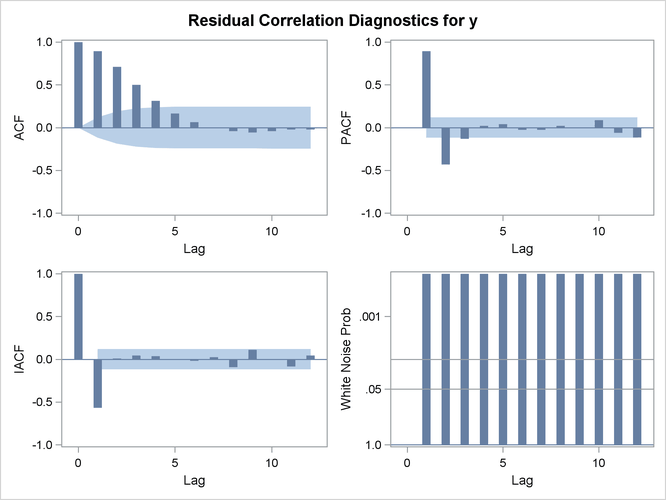The residual correlation analysis suggests an AR(2) model for the noise part of the model. The ESTIMATE statement results for the final transfer function model with AR(2) noise are shown in Output 7.3.11.

Output 7.3.11: Estimation Output of the Final Model

Conditional Least Squares Estimation
Parameter Estimate Standard Error t Value Approx
Pr > |t|
Lag Variable Shift
MU 53.26304 0.11929 446.48 <.0001 0 y 0
AR1,1 1.53291 0.04754 32.25 <.0001 1 y 0
AR1,2 -0.63297 0.05006 -12.64 <.0001 2 y 0
NUM1 -0.53522 0.07482 -7.15 <.0001 0 x 3
NUM1,1 0.37603 0.10287 3.66 0.0003 1 x 3
NUM1,2 0.51895 0.10783 4.81 <.0001 2 x 3
DEN1,1 0.54841 0.03822 14.35 <.0001 1 x 3

 Constant Estimate 5.32942 0.058828 0.242544 8.29281 34.0061 291

Output 7.3.12: Residual Analysis of the Final Model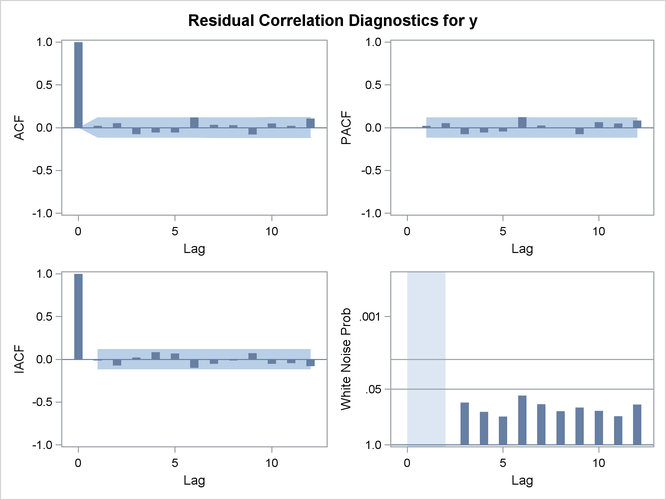Output 7.3.13: Model Summary of the Final Model

Model for variable y
Estimated Intercept 53.26304

Autoregressive Factors
Factor 1: 1 - 1.53291 B**(1) + 0.63297 B**(2)

Input Number 1
Input Variable x
Shift 3

Numerator Factors
Factor 1: -0.5352 - 0.37603 B**(1) - 0.51895 B**(2)

Denominator Factors
Factor 1: 1 - 0.54841 B**(1)